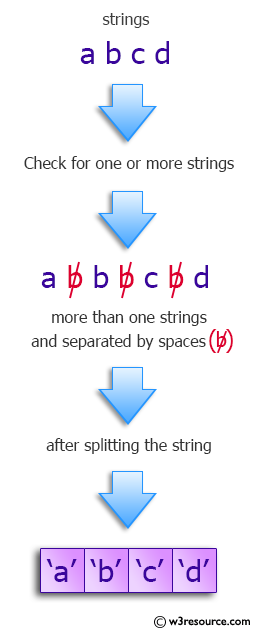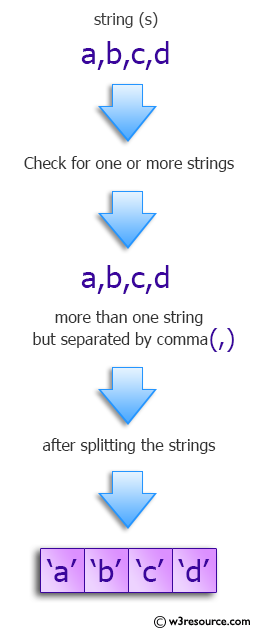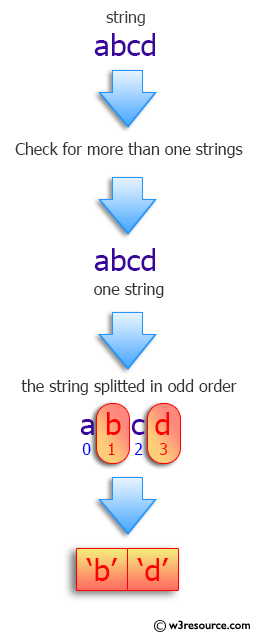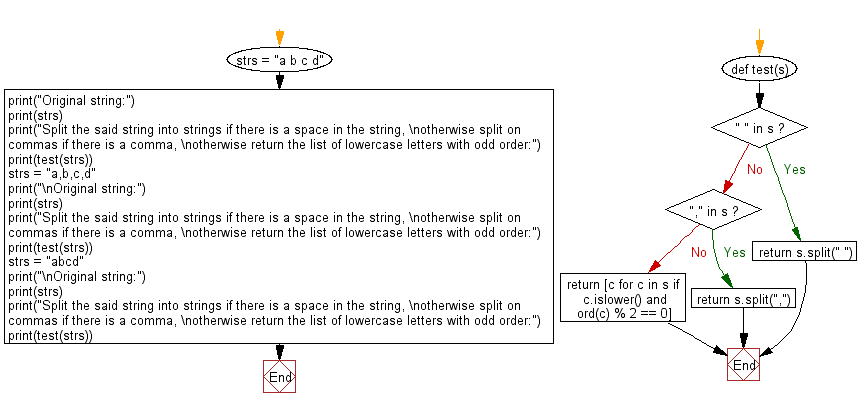﻿ Python: Split a string into strings if there is a space in the string, otherwise split on commas, otherwise the list of lowercase letters with odd order - w3resource# Python: Split a string into strings if there is a space in the string, otherwise split on commas, otherwise the list of lowercase letters with odd order

## Python Programming Puzzles: Exercise-19 with Solution

Write a Python program to split a given string (s) into strings if there is a space in the string, otherwise split on commas if there is a comma, otherwise return the list of lowercase letters with odd order (order of a = 0, b = 1, etc.)

```Input:
a b c d
Split the said string into strings if there is a space in the string,
otherwise split on commas if there is a comma,
Output:
['a', 'b', 'c', 'd']

Input:
a,b,c,d
Split the said string into strings if there is a space in the string,
otherwise split on commas if there is a comma,
Output:
['a', 'b', 'c', 'd']

Input:
abcd
Split the said string into strings if there is a space in the string,
otherwise split on commas if there is a comma,
Output:
['b', 'd']
```

Pictorial Presentation:Sample Solution:

Python Code:

``````#License: https://bit.ly/3oLErEI

def test(s):
if " " in s:
return s.split(" ")
if "," in s:
return s.split(",")
return [c for c in s if c.islower() and ord(c) % 2 == 0]

strs = "a b c d"
print("Original string:")
print(strs)
print("Split the said string into strings if there is a space in the string, \notherwise split on commas if there is a comma, \notherwise return the list of lowercase letters with odd order:")
print(test(strs))
strs = "a,b,c,d"
print("\nOriginal string:")
print(strs)
print("Split the said string into strings if there is a space in the string, \notherwise split on commas if there is a comma, \notherwise return the list of lowercase letters with odd order:")
print(test(strs))
strs = "abcd"
print("\nOriginal string:")
print(strs)
print("Split the said string into strings if there is a space in the string, \notherwise split on commas if there is a comma, \notherwise return the list of lowercase letters with odd order:")
print(test(strs))
``````

Sample Output:

```Original string:
a b c d
Split the said string into strings if there is a space in the string,
otherwise split on commas if there is a comma,
otherwise return the list of lowercase letters with odd order:
['a', 'b', 'c', 'd']

Original string:
a,b,c,d
Split the said string into strings if there is a space in the string,
otherwise split on commas if there is a comma,
otherwise return the list of lowercase letters with odd order:
['a', 'b', 'c', 'd']

Original string:
abcd
Split the said string into strings if there is a space in the string,
otherwise split on commas if there is a comma,
otherwise return the list of lowercase letters with odd order:
['b', 'd']
```

Flowchart:## Visualize Python code execution:

The following tool visualize what the computer is doing step-by-step as it executes the said program:

Python Code Editor :

Have another way to solve this solution? Contribute your code (and comments) through Disqus.

What is the difficulty level of this exercise?

Test your Programming skills with w3resource's quiz.

﻿

## Python: Tips of the Day

Decapitalizes the first letter of a string:

Example:

```def tips_decapitalize(s, upper_rest=False):
return s[:1].lower() + (s[1:].upper() if upper_rest else s[1:])
print(tips_decapitalize('PythonTips'))
print(tips_decapitalize('PythonTips', True))
```

Output:

```pythonTips
pYTHONTIPS
```